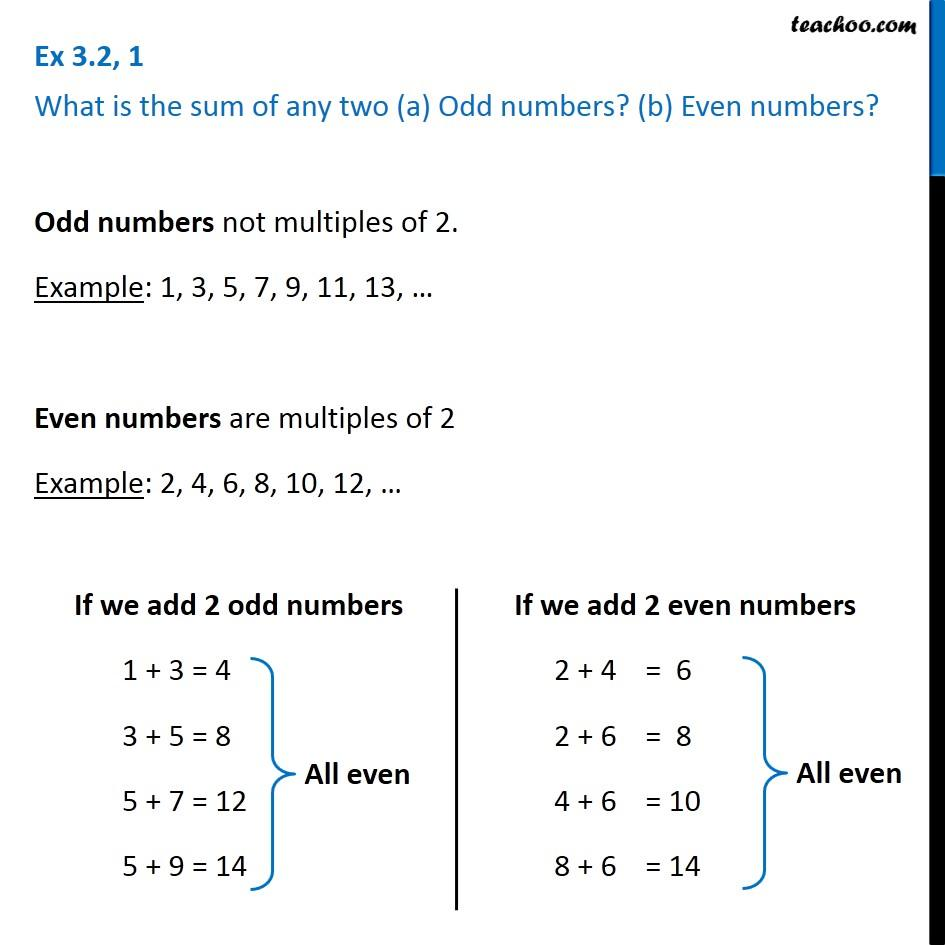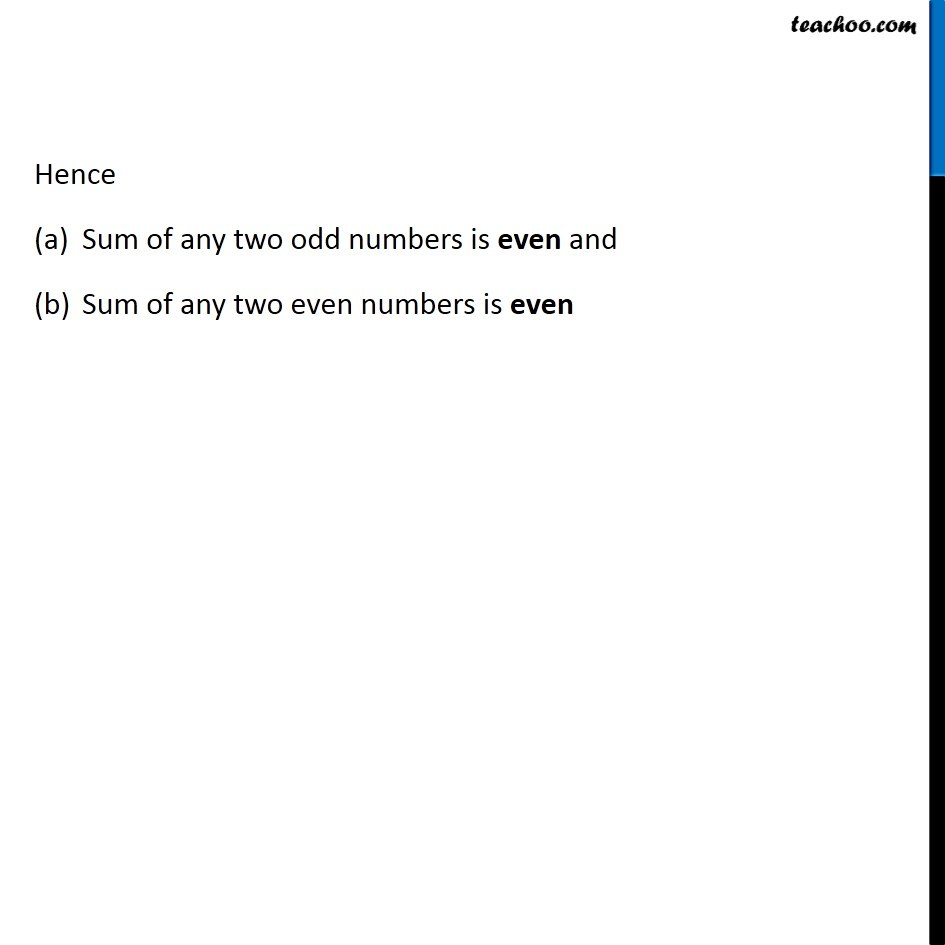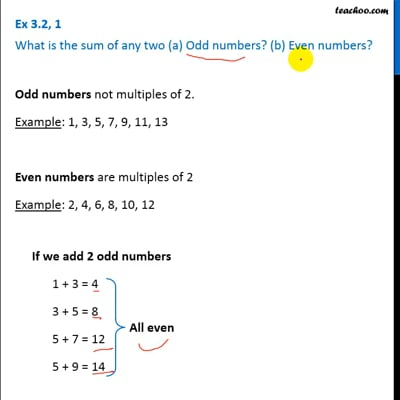Sum of prime numbers

Chapter 3 Class 6 Playing with Numbers
Concept wiseThis video is only available for Teachoo black users

Introducing your new favourite teacher - Teachoo Black, at only ₹83 per month

### Transcript

Ex 3.2, 1 What is the sum of any two (a) Odd numbers? (b) Even numbers?Odd numbers not multiples of 2. Example: 1, 3, 5, 7, 9, 11, 13, Even numbers are multiples of 2 Example: 2, 4, 6, 8, 10, 12, If we add 2 odd numbers 1 + 3 = 4 3 + 5 = 8 5 + 7 = 12 5 + 9 = 14 If we add 2 even numbers 2 + 4 = 6 2 + 6 = 8 4 + 6 = 10 8 + 6 = 14 Hence Sum of any two odd numbers is even and Sum of any two even numbers is even